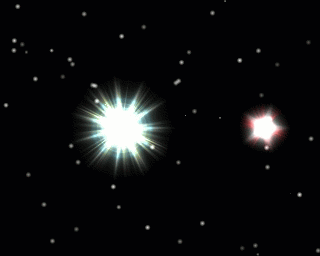# Binary starsImagine two stars $A$ and $B$ forming a binary star system, where they revolve around a common point in space under their mutual gravitational attraction alone. If $A$ is heavier than $B$ and they both revolve in circular orbits, then which star will have a smaller orbital radius?

×